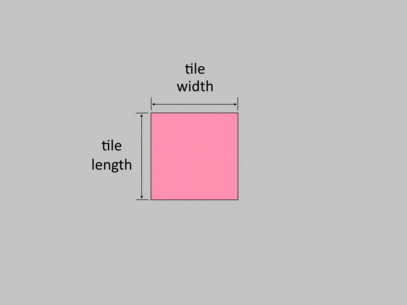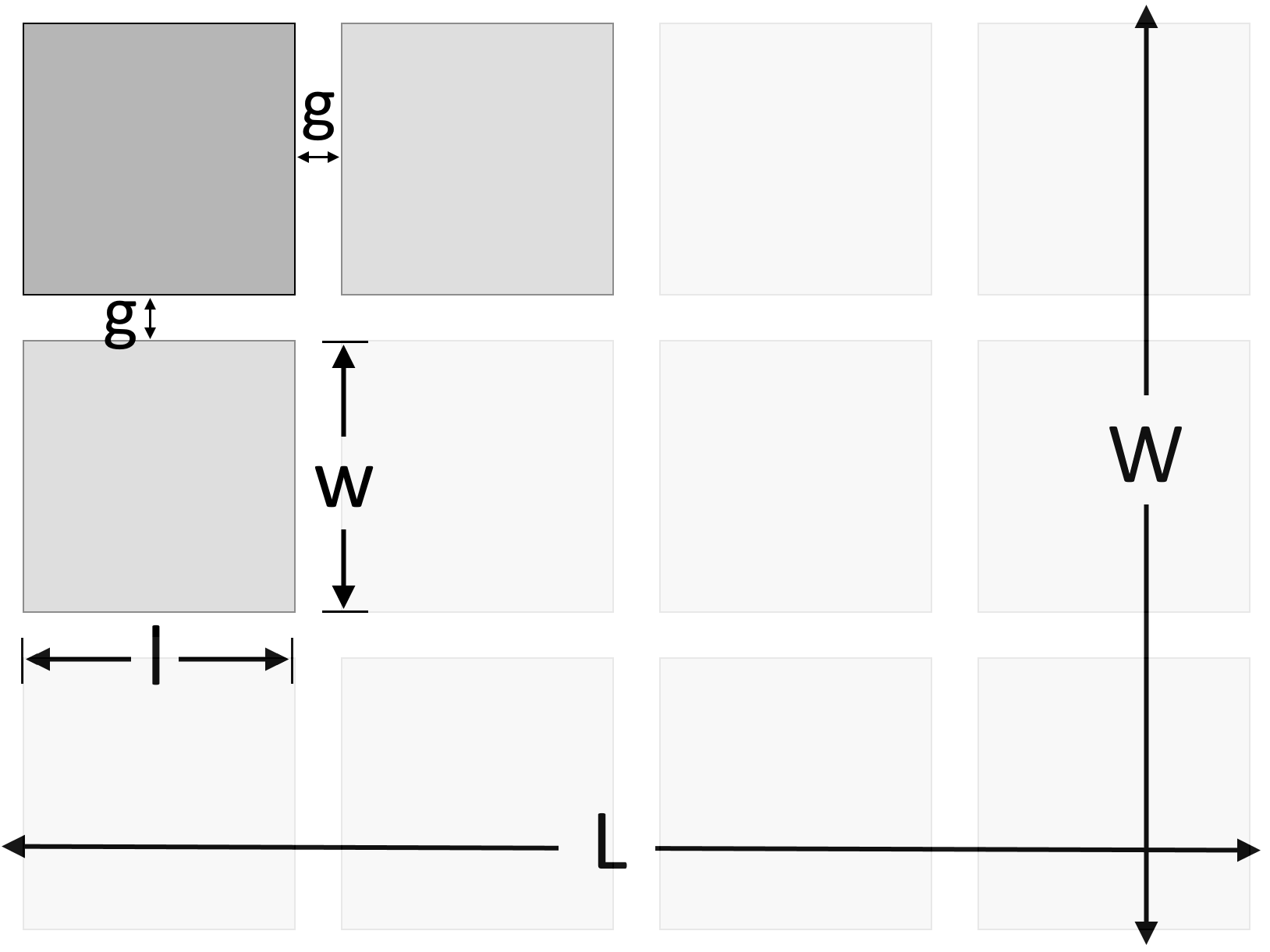# Grout Calculator

Created by Kenneth Alambra
Reviewed by Dominik Czernia, PhD
Last updated: Nov 03, 2023

If you're wondering "How much grout do I need for my tiling project?" then this grout calculator, or more specifically, tile grout calculator, is for you.

In this calculator, you will learn what tile grout or tile gap filler is and how to calculate cement grout volume for any area of floor or wall, tile size, and tile gap. Keep on reading to learn more.

💡 This tool, together with our tile calculator and our thinset calculator, is part of our tiling tools set you can use for worry-free tiling projects. Check them out to know how many tiles you'll need and how much thinset you'll need to adhere your tiles to your substrate.

## What is tile grout?

Tile grout is a material used to fill in the gaps between the tiles to avoid dirt and liquid from coming in. When installing tiles, we place the tiles with gaps between each other to decrease the chances of future cracking or lifting off of tiles due to thermal or structural deformation of the floor or wall, tile expansion, or tile movement. Using tile grout also adds to the aesthetics of a tile installation, especially of ceramic tiles, like in this picture:Grout, in general, is a composite material which is typically a mixture of cement, lime, and water, and can also contain sand just like concrete and mortar. You can check our cement calculator to learn more about these other mixtures. But you can also visit our concrete calculator and our mortar calculator if you want to read more about their applications.

Some grouts are made of epoxy and polymers; these types of grout are best for areas that need high-resistance to moisture and chemicals. With that said, we can either get dry pre-mixed cementitious grout in bags or in 2-part bottles with epoxy hardener and resin.

## How do you calculate cement grout volume?

To calculate the volume of grout we need, we simply need to determine the total volume of the gap between our tiles. In this calculator, we utilized the ratio $R$ of the area of a single tile to the area of a single tile with half of the gap surrounding it, as shown below:

$R = \frac{l \times w}{(l + g) \times (w + g)},$

where:

• $l$ – Length of the tiles to be installed;
• $w$ – Width of the tiles to be installed; and
• $g$ – Gap width or the tile spacing that needs grouting.

Using this ratio, we can then find the total area covered by the tile grout using the equation below:

$\small \text{grout area} = A_\text{surface}\! -\! A_\text{surface}\! \times\! R,$

where:

• $\text{grout area}$ – Total area that needs grouting; and
• $A_\text{surface}$ – Total area of the tiling project.

By utilizing ratio $R$, we get the corresponding total area of tiles with grout while considering a gap of width $g$ between each tile, as well as a gap of half of $g$ around the perimeter of the area, as you can see in this animation:To finally obtain the volume of the grout needed, we just need to multiply the grout area by the depth of the gap, like in this equation:

$\small \text{grout volume}\! =\! \text{grout area}\! \times\! \text{gap depth}$

We can also use this grout volume formula for epoxy-type tile grout. All you have to do is convert the volume unit used into liquid volume units such as milliliters or ounces.

## How do you use our grout calculator?

To use our grout calculator, enter the dimensions of the area that needs tiling, the dimensions of your tiles, and your desired gap width and depth. Our grout calculator will instantly show you the amount of grout you would need for your project.

We have also included an estimated number of bags of dry pre-mixed grout you would need for your project. In our calculator, we set the default value for the average density of grout to be 1,600 kg/m³ (100 lb/ft³) and use a 1:1 ratio of water to dry pre-mixed grout (as indicated by the 50% in the dry material percentage field). You can access and edit these fields in the Advanced mode of our grout calculator.

Tiling and grouting are just a few of the things we can do to improve the looks of our homes, offices, and even swimming pools. If you want to learn more about other flooring or wall coverings, consider checking out our brick calculator or our vinyl siding calculator, to name some.

## FAQ

### How much grout do I need per square foot?

The amount of grout per square foot depends on tile size, spacing, and the width and depth of the gap.

1. First, calculate the area that will need grouting:

Grout area = Aₛ − Aₛ × R

R = Lₜ × Wₜ / ((Lₜ + Gₜ) × (Wₜ + Gₜ)).

Where:

• Aₛ - The surface area for tiling and grouting.
• Lₜ - Length of the tile;
• Wₜ - Width of the tile; and
• Gₜ - The gap width or tile spacing that needs grouting.
1. Then calculate the grout volume:

Grout volume = Grout area × gap depth

### How much grout do I need for a 50 square feet backsplash?

You need 0.02626 ft³ of grout for a 50 ft² backsplash. Assuming the area covered by a single tile is 1 ft². And your choice of tile gap is 5mm.

### How do I calculate how much grout I need?

To calculate the grout needed, follow the steps below:

1. Note down the total floor area that you want to tile as Aₛ.

2. Measure your tile length and width as Lₜ and Wₜ.

3. Choose the gap you want between the tiles as Gₜ.

4. Then, use the following formula to find the total grout area.

Grout area = Aₛ - Aₛ × (Lₜ × Wₜ / ((Lₜ + Gₜ) × (Wₜ + Gₜ)))

5. Now multiply the grout area by your desired gap depth to get the required volume.

### How much grout do I need for a 12 × 24 cm tile?

You need about 33 cm³ of grout for a tile with length 12 cm and width 24 if your total surface area is 290 cm², with the border gap 1 cm around the tile.

You can use the following formula:
Grout = Aₛ - Aₛ × (Lₜ × Wₜ / ((Lₜ + Gₜ) × (Wₜ + Gₜ)))

Where:

• Aₛ - Area of the surface;
• Lₜ - Length of the tile;
• Wₜ - Width of the tile; and
• Gₜ - Tile gap.
Kenneth Alambra
Area to be tiledLength of the area (L)
ft
Width of the area (W)
ft
Total area to be tiled
ft²
Tile details
Length of tile (l)
in
Width of tile (w)
in
Area covered by a single tile
in²
Gap details
Gap width (g)
in
Gap depth
in
Output value
Grout volume
cu in
Weight per bag
lb
Bags
bags
People also viewed…

### Rip rap

Find the average diameter of rocks to use for your embankment with this rip rap calculator.

### Sunbathing

Do you always remember to put on sunscreen before going outside? Are you sure that you use enough? The Sunbathing Calculator ☀ will tell you when's the time to go back under an umbrella not to suffer from a sunburn!

### Titration

Use our titration calculator to determine the molarity of your solution.

### Tonnage

This tonnage calculator can determine the weight in tons of certain aggregate materials depending on their unit weights and the volume they occupy.